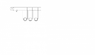# Finding diameter in a piping system

theone

## Homework Statement

I am trying to find the diameter of two types of pipe in this system

## Homework Equations

## \frac{p_1}{y} + \frac{V_1}{2g} + z_1 = \frac{p_2}{γ} + \frac{V_2}{2g} + z_2 + \frac{V_2}{2g} [ \frac{fL}{D} + K ]##

## The Attempt at a Solution

The only flow rate I'm given is the one where each reactor would be at maximum heat load. Should I use that in the equation above to get the diameters?

#### Attachments

•Untitled.png
6.6 KB · Views: 350

Mentor
Can you please provide an actual problem statement? Put yourself in our place. Did you really think we could figure out what you were talking about?

Chet

theone
Can you please provide an actual problem statement? Put yourself in our place. Did you really think we could figure out what you were talking about?

Chet

edit: actually its ok, I got it now

#### Attachments

• Assignment 1 F15.pdf
107.5 KB · Views: 295
Last edited: